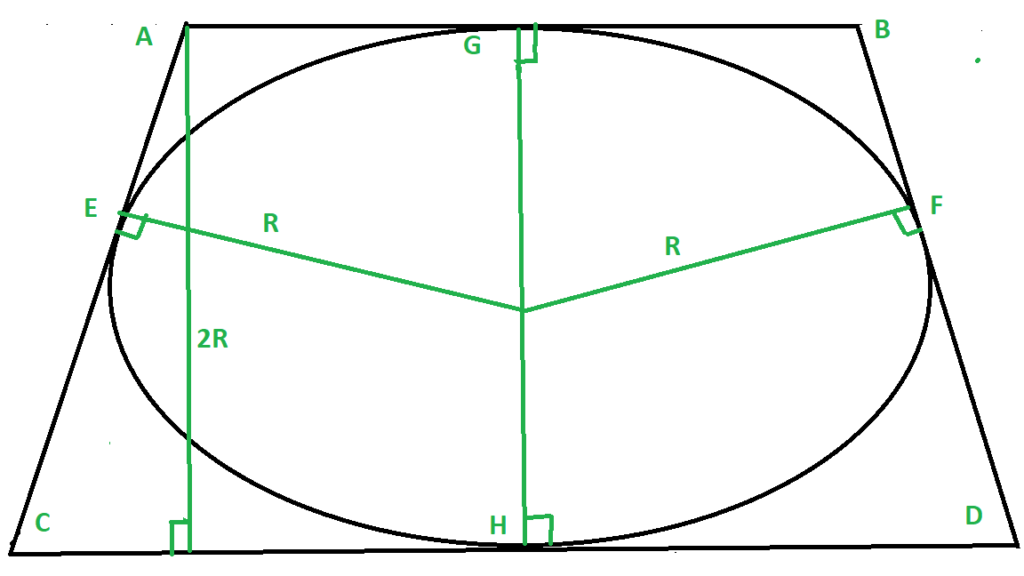# Area of circle inscribed in a Isosceles Trapezoid

• Last Updated : 07 Sep, 2021

Given two bases of the isosceles trapezoid ABCD as a and b, the task is to find the area of circle inscribed in this trapezoidAttention reader! Don’t stop learning now. Get hold of all the important DSA concepts with the DSA Self Paced Course at a student-friendly price and become industry ready.  To complete your preparation from learning a language to DS Algo and many more,  please refer Complete Interview Preparation Course.

In case you wish to attend live classes with experts, please refer DSA Live Classes for Working Professionals and Competitive Programming Live for Students.

Examples:

```Input: a = 10, b = 30
Output: Area = 235.57

Input: a = 20, b = 36
Output: Area = 565.38```

Derivation: Given a circle inscribed in trapezium ABCD (sides AB = n and CD = m), we need to find out the height of the trapezium i.e., (AL), which is half of the radius of the circle to find the area of the circle.For finding the height of circle we do following operation.

1. The circle will always touch the sides of trapezium at their midpoints, Say the midpoints of AB, BD, CD, AC are G, F, H, E, and join them with the center of the circle.
2. Now from Symmetry, we can see that
```AG = AE = n/2,
EC = CG = m/2,
HD = DF = n/2,
GB = FB = m/2```
3. Now in Triangle ACL apply the Pythagoras theorem.
```Hypotenuse AC = m/2 + n/2
Base CL = CH - AG = m/2 - n/2

we get
Perpendicular AL = Square_root(m * n)```
4. Therefore the height of the Trapezium = AL = Square_Root(Product of given sides)
5. Now the radius of the circle is simple half of the height and hence the area can be calculated easily.

Approach:

1. Find the height of the trapezoid as (square_root( m * n )).
2. Find the radius of the incircle
```R = height / 2
= square_root(m * n) / 2```
3. Now find the area of the circle
```= Pi * R2
= ( 3.141 * m * n ) / 4```

Below is the implementation of the above approach:

## C++

 `// CPP implementation to find``// the rea of the circle``// inscribed in a trapezoid``// having non- parallel sides m, n``#include``using` `namespace` `std;`` ` `// Function to find area of circle``// inscribed in a trapezoid``// having non- parallel sides m, n``double` `area_of_circle(``int` `m, ``int` `n)``{``    ``// radius of circle by the``    ``// formula i.e. root( m * n) / 2``    ``// area of circle = (3.141 ) * ( R ** 2 )`` ` `    ``int` `square_of_radius = ( m * n ) / 4;``    ``double` `area = ( 3.141 * square_of_radius );``    ``return` `area;``}`` ` `// Driver Code``int` `main(){``    ``int` `n = 10;``    ``int` `m = 30;``    ``cout << (area_of_circle(m, n));``}`` ` `// This code is contributed by mohit kumar 29`

## Java

 `// Java Program to find ``// the rea of the circle ``// inscribed in a trapezoid ``// having non- parallel sides m, n ``class` `GFG ``{ ``     ` `    ``// Function to find area of circle ``    ``// inscribed in a trapezoid ``    ``// having non- parallel sides m, n ``    ``static` `double` `area_of_circle(``int` `m, ``int` `n) ``    ``{ ``        ``// radius of circle by the ``        ``// formula i.e. root( m * n) / 2 ``        ``// area of circle = (3.141 ) * ( R ** 2 ) ``     ` `        ``int` `square_of_radius = ( m * n ) / ``4``; ``        ``double` `area = ( ``3.141` `* square_of_radius ); ``        ``return` `area; ``    ``} ``     ` `    ``// Driver code ``    ``public` `static` `void` `main (String[] args) ``    ``{ ``        ``int` `n = ``10``; ``        ``int` `m = ``30``; ``        ``System.out.println(area_of_circle(m, n)); ``    ``} ``} `` ` `// This code is contributed by Yash_R`

## Python3

 `# Python 3 implementation to find ``# the rea of the circle ``# inscribed in a trapezoid``# having non- parallel sides  m, n`` ` ` ` `# Function to find area of circle ``# inscribed in a trapezoid``# having non- parallel sides  m, n``def` `area_of_circle(m, n):``    ``# radius of circle by the``    ``# formula i.e. root( m * n) / 2``    ``# area of circle = (3.141 ) * ( R ** 2 )``     ` `    ``square_of_radius ``=` `( m ``*` `n ) ``/` `4``    ``area ``=` `( ``3.141` `*` `square_of_radius )``    ``return` `area`` ` `# Driver Code ``if` `__name__``=``=``'__main__'``: ``    ``n ``=` `10``    ``m ``=` `30``    ``print``(area_of_circle(m, n))`

## C#

 `// C# Program to find ``// the rea of the circle ``// inscribed in a trapezoid ``// having non- parallel sides m, n ``using` `System; `` ` `class` `GFG ``{ ``     ` `// Function to find area of circle ``// inscribed in a trapezoid ``// having non- parallel sides m, n ``static` `double` `area_of_circle(``int` `m, ``int` `n) ``{ ``    ``// radius of circle by the ``    ``// formula i.e. root( m * n) / 2 ``    ``// area of circle = (3.141 ) * ( R ** 2 ) `` ` `    ``int` `square_of_radius = ( m * n ) / 4; ``    ``double` `area = ( 3.141 * square_of_radius ); ``    ``return` `area; ``} `` ` `// Driver code ``public` `static` `void` `Main () ``{ ``    ``int` `n = 10; ``    ``int` `m = 30;``    ``Console.WriteLine(area_of_circle(m, n)); ``} ``} `` ` `// This code is contributed by Sanjit_Prasad`

## Javascript

 ``
Output:
`235.575`

My Personal Notes arrow_drop_up